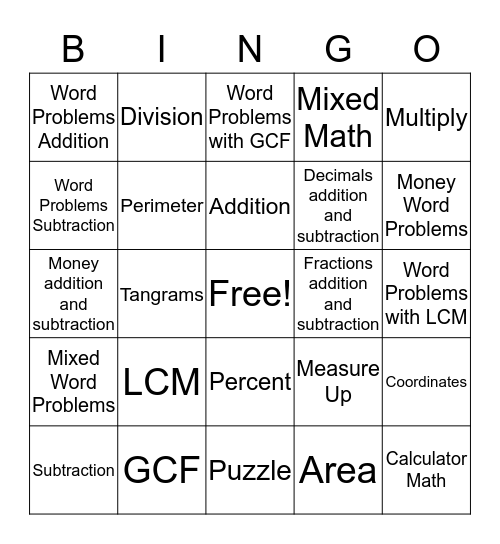# Math BingoThis bingo card has a free space and 24 words: LCM, GCF, Addition, Subtraction, Multiply, Word Problems Addition, Word Problems Subtraction, Division, Fractions addition and subtraction, Percent, Decimals addition and subtraction, Money addition and subtraction, Measure Up, Coordinates, Perimeter, Area, Word Problems with LCM, Word Problems with GCF, Money Word Problems, Tangrams, Calculator Math, Mixed Word Problems, Mixed Math and Puzzle.

## Play Online